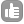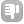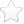1Hello all, iam trying to key in the correct value for nd0 for a custom written pulse program. In doing so i ran into confusion as the pulse sequence adopt constant time approach. I read from the Bruker maual that for F1(indirect dimension), SW = 1/(in0*nd0), where SW:spectral width, in0: incremental delay for d0 (t1 increment), nd0: number of d0 delays. Based on this formula, i assume that given a SW value, in0 will be calculated by Bruker software for a defined nd0 and vice-versa (SW being calculated from the in0 value). So i can guess the in0 and nd0 for the following cases would be, A) |<-----------------------------------t1------------------------------------->| `````` (in0 = t1(dwell time), nd0 =1) `````` B) |<--------------t1/2---------------><------------------t1/2-------------->| `````` (in0 = t1(dwell time)/2, nd0 =2) `````` C) |<-----t1/4-----><-----t1/4------><------t1/4-----><-----t1/4------>| `````` (in0 = t1(dwell time)/4, nd0 =4) `````` My problem is, the experiment is an interleaved one with type (C) experiment performed first followed by type (B) experiment. Since i cannot set two different values for d0 and nd0, iam not sure how to proceed. Will it be fine if i altogether ignore d0 and use 6 different delays (e.g d20 to d25) with its respective increments (in20 to in25), and do not worry about nd0 value at all? Will it cause any problem while running the experiment? asked Jun 19 '12 at 11:14Jana 312Dear Jana, nd0 is mainly used to calculate a correct in0 from the value of SWH in the indirect dimension or vice versa. If you set in0 manually to the correct value and nd0 is wrong you will end up with an incorrect axis. There are multiple solutions for your problem. If you want to set nd0 to 4 then you can just use the delay d0 twice back to back in the part of the sequence which follows example B. For example you can write d0 - pulse - d0 - pulse - d0 - pulse - d0 for the type C part and d0 - d0 - pulse -d0 -d0 for the type B part. You can also use different delays, but then you have to make sure that in0, nd0 and SWH look correct, to give you the right axis in the spectrum. What version of software are you using? Clemens answered Jun 23 '12 at 03:31Dear Clemens, Thank you for that clever trick on back to back d0 usage. Iam currently using Topspin 3.0 for data acquisition in Bruker and use NMRPipe 9 for data processing. - Jana (Jun 23 '12 at 12:47) Dear Jana, in Topspin 3.0 nd0 is no longer used. Based on SW in a dimension the parameter inf(n) is calculated and you recalculate it for use in a pulsprogram with the equation "in0=inf1/2" when case B is used or "in0=inf1/4" when C is used. Let's take this off-line write me at cga@bruker.com - Clemens Anklin (Jun 30 '12 at 14:28)

 toggle preview

Tags:

×6
×1
×1
×1
×1

Asked: Jun 19 '12 at 11:14

Seen: 5,248 times

Last updated: Jun 30 '12 at 14:28